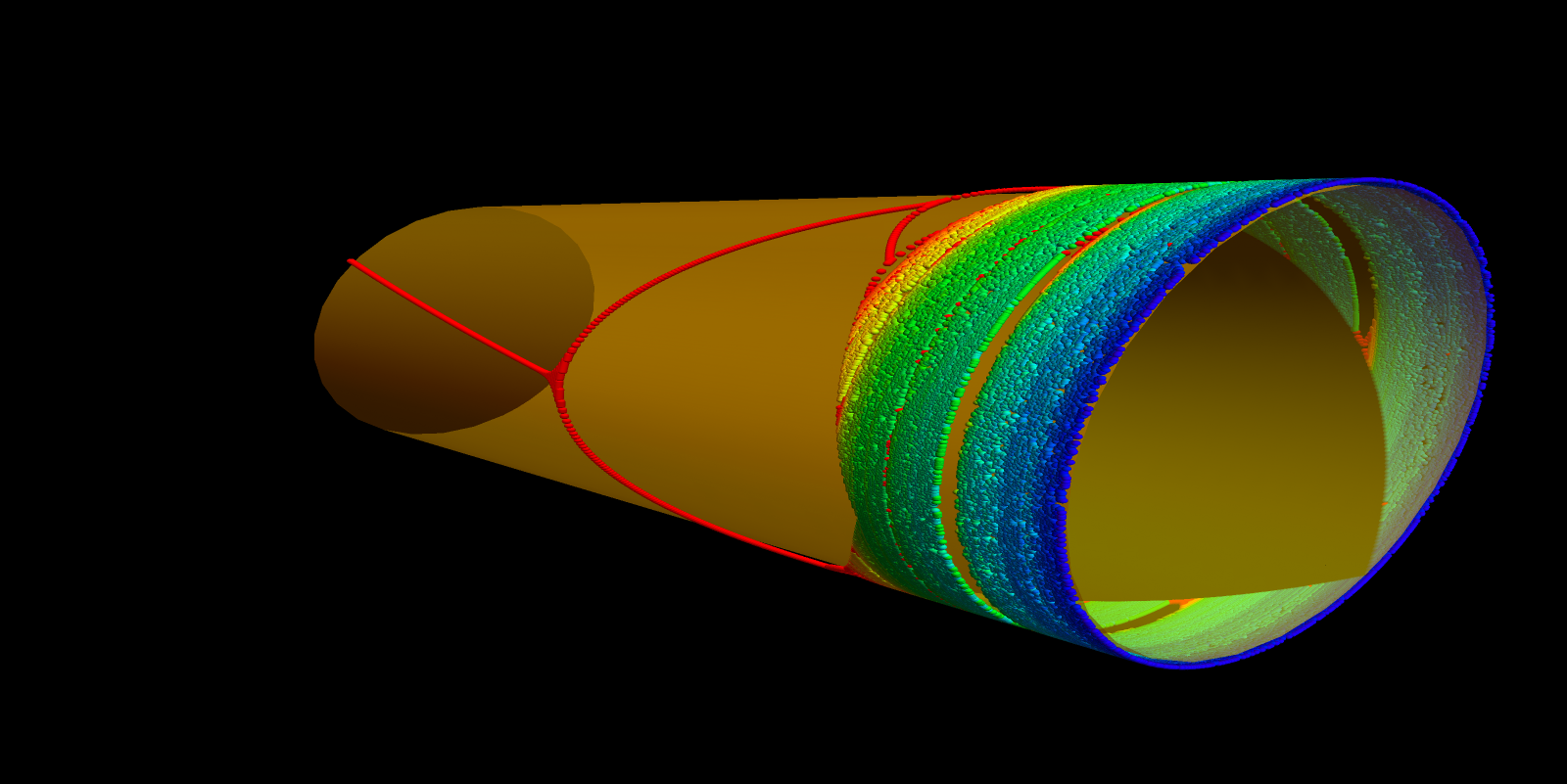# Feigenbaum

Mitchell Feigenbaum, who died on June 30 2019, discovered about 50 years ago an important universality: there are quantities in dynamics which do not depend on the rule which produce the dynamics. Let us explain this with the circle Tc(x) = c sin( π x) which maps the interval [0,1] into itself and can also be seen as a map on the circle X=R/Z in which the point 0 and 1 are identified forming a circle. Now, if c is very small, there is just one attractor 0. What Feigenbaum discovered with experiments done on a calculator is that the bifurcation values ck at which the periodic attractors change accumulate in a universal way. Universal means that it does not matter, what map we take. For T(x) = 4c x(1-x) it would show a similar bifurcation structure near the threshold to chaos (which can be defined as a situation, where the attractor is no more finite.) A more precise definition of chaos is ``sensitive dependence of initial condition" meaning that the Lyapunov exponent L(c) = liminfn → ∞ log|(Tcnx)'|/n is positive when integrated over all x in X). Here is a picture of one parameter circle map f_c(x) = c sin(pi x), where c ranges from 0.55 to 1. If you slice the cylinder at some hight, you see the corresponding atractor of the map f_c. At c=1, there is chaos. The route to chaos has come through bifurcations, which show universality.Here are pictures of Feigenbaum (parts of photographs on Washington post and NYT):